# Chapter 11: Algebra NCERT Solutions Exercise 11.1,11.2,11.3

In this page we have NCERT Solutions for Class 6 Maths Chapter 11: Algebra Exercise 11.1,11.2,11.3. Hope you like them and do not forget to like , social share and comment at the end of the page.
Topics of Use of variables in common rules,Expressions with variables,Using expressions practically,What is an equation?,Solution of an equation is deleted from this chapter as per rationalized syllabus. So you may ignore the exercise 11.2. and 11.3

## Exercise 11.1

Question 1
Find the rule which gives the number of matchsticks required to make the following matchstick patterns. Use a variable to write the rule.
(a) A pattern of letter T as T
(b) A pattern of letter Z as Z
(c) A pattern of letter U as U
(d) A pattern of letter V as V
(e) A pattern of letter E as E
(f) A pattern of letter S as S
(g) A pattern of letter A as A
a) It is apparent from the letter T that 2 matchsticks will be required to create one T.
If we need to create 2 T, then we will require 2×2=4 match sticks, similarly for 3, 2×3=6
So if n is the number of T’s, the matchstick required will be 2×n=2n
b) It is apparent from the letter Z that 3 matchsticks will be required to create one Z.
If we need to create 2 Z, then we will require 3×2=6 match sticks, similarly for 3, 3×3=9
So if n is the number of Z’s, the matchstick required will be 3×n=3n
c) It is apparent from the letter U that 3 matchsticks will be required to create one U.
If we need to create 2 U, then we will require 3×2=6 match sticks, similarly for 3, 3×3=9
So if n is the number of U’s, the matchstick required will be 3×n=3n
d) It is apparent from the letter V that 2 matchsticks will be required to create one V.
If we need to create 2 V, then we will require 2×2=4 match sticks, similarly for 3, 2×3=6
So if n is the number of V’s, the matchstick required will be 2×n=2n
e) It is apparent from the letter E that 5 matchsticks will be required to create one E.
If we need to create 2 E, then we will require 5×2=10 match sticks, similarly for 3, 5×3=15
So if n is the number of E’s, the matchstick required will be 5×n=5n
f)  It is apparent from the letter S that 5 matchsticks will be required to create one S
If we need to create 2 S, then we will require 5×2=10 match sticks, similarly for 3, 5×3=15
So if n is the number of S’s, the matchstick required will be 5×n=5n
g)  It is apparent from the letter A that 6 matchsticks will be required to create one A.
If we need to create 2 A, then we will require 6×2=12 match sticks, similarly for 3, 6×3=18
So if n is the number of A’s, the matchstick required will be 6×n=6n

Question 2
We already know the rule for the pattern of letters L, C and F. Some of the letters from some of the letters out of (a) T, (b) Z, (c) U, (d) V, (e) E, (f) S, (g) R give us the same rule as that given by L. Which are these? Why does this happen?
a) And (d), that is, letters T and V have the same pattern rule as for letters L, C and F as the number of matchsticks required to make the letter is 2.

Question 3
Cadets are marching in a parade. There are 5 cadets in a row. What is the rule which gives the number of cadets, given the number of rows? (Use n for the number of rows.)
Number of rows = n
Now Number of cadets in a row = 5
Total number of cadets is given by the rule = Number of cadets in a row × Number of rows
=5 × number of rows
= 5n

Question 4
If there are 50 mangoes in a box, how will you write the total number of mangoes in terms of the number of boxes? (Use b for the number of boxes.)
Number of boxes is given by the variable =b
Number of mangoes in one box = 50
So, total number of mangoes is given by the rule =Number of mangoes in a box × number of boxes
= 50 × number of boxes
= 50b
Question 5
The teacher distributes 5 pencils per student. Can you tell how many pencils are needed, given the number of students? (Use s for the number of students.)
Number of students is given by the variable= s
Number of pencils given to one student = 5
So, total number of pencils is given by the rule = Number of pencils per one students × number of students
=5 × number of students
= 5s
Question 6
A bird flies 1 kilometer in one minute. Can you express the distance covered by the bird in terms of its flying time in minutes? (Use t for flying time in minutes.)
Flying time in minutes is given by the variable= t
Distance fled by the bird in one minute = 1 km
Distance covered by the bird in minutes is given by the rule = distance covered in 1 min ×       flying time
= 1 × t
= t km.

Question 7
Radha is drawing a dot Rangoli (a beautiful pattern of lines joining dots with chalk powder. She has 9 dots in a row. How many dots will her Rangoli have for r rows? How many dots are there if there are 8 rows? If there are 10 rows?
Number of dots in a row = 9
Number of rows is a variable= r
Total number of dots in r rows = number of dots in one row × number of rows
= 9r
Total number of dots in 8 rows = 9 × 8 = 72
Total number of dots in 10 rows    = 9 × 10 = 90

Question 8
Leela is Radha’s younger sister. Leela is 4 years younger than Radha. Can you write Leela’s age in terms of Radha’s age? Take Radha’s age to be x years.
Given
Leela is Radha’s younger sister  and she  is 4 years younger than Radha
So Leela age = Radha’s age – 4
Leela’s age = x – 4 years

Question 9
Number of laddus mother gave away = l.
Number of laddus remaining = 5
= Number of laddus mother gave away + Number of laddus remaining
=l + 5.

Question 10
Oranges are to be transferred from larger boxes into smaller boxes. When a large box is emptied, the oranges from it fill two smaller boxes and still 10 oranges remain outside. If the number of oranges in a small box are taken to be x, what is the number of oranges in the larger box?
Number of oranges in the small box = x
When a large box is emptied, the oranges from it fill two smaller boxes and still 10 oranges remain outside.
So Number of oranges in the larger box = Number of oranges in two small boxes + 10 oranges
= x + x + 10 = 2x + 10

Question 11
(a) Look at the following matchstick pattern of squares. The squares are not separate. Two neighboring squares have a common matchstick. Observe the patterns and find the rule that gives the number of matchsticks in terms of the number of squares. (Hint: if you remove the vertical stick at the end, you will get a pattern of Cs.)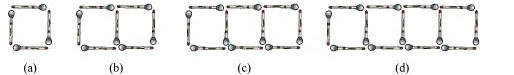(b) The given figure gives a matchstick pattern of triangles. Find the general rule that gives the number of matchsticks in terms of the number of triangles.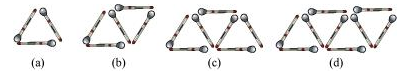a) It can be observed that number of matchsticks in this pattern is in the way
 Options a) b) c) d) Number of matchsticks 4 7 10 13 Number of squares 1 square 2 square 3 square 4 square Pattern we can look out 3(number of squares) +1 3(number of squares) +1 3(number of squares) +1 3(number of squares) +1
Let ‘s take variable x denotes the number of squares
Then Number of matchsticks required can be given as
=3x+1
b)
a) It can be observed that number of matchsticks in this pattern is in the way
 Options a) b) c) d) Number of matchsticks 3 5 7 9 Number of triangles 1 triangle 2 triangles 3 triangles 4 triangles Pattern we can look out 2(number of triangles) +1 2(number of triangles) +1 2(number of triangles) +1 2(number of triangles) +1
Let ‘s take variable x denotes the number of triangles
Then Number of matchsticks required can be given as
=2x+1

## Exercise 11.2

Question 1
The side of an equilateral triangle is shown by l. Express the perimeter of the equilateral triangle using l.
We know that equilateral triangle has three equal sides, l Perimeter of equilateral triangle
= the sum of the three sides
= l + l + l =3 l
Question 2
The Side of a regular hexagon (see the given figure) is denoted by l. Express the perimeter of the hexagon using l.
(Hint: A regular hexagon has all its six sides equal in length.)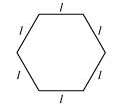We know that regular hexagon has six equal sides, l Perimeter of Hexagon
= the sum of the six sides
= $l + l+ l+ l+ l+ l$ =$6 l$

Question 3
A cube is a three-dimensional figure as shown in the given figure. It has six faces and all of them are identical squares. The length of an edge of the cube is given by l. Find the formula for the total length of the edges of a cube.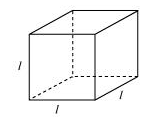Cube has twelve equal sides, l
Total length of the cube
= the sum of the twelve sides
= $l+ l+ l+ l+ l+ l+ l+ l+ l+ l+ l+ l$ =$12 l$

Question 4
The diameter of a circle is a line which joins two points on the circle and also passed through the center of the circle. (In the adjoining figure AB is a diameter of the circle; C is its center.) Express the diameter of the circle (d) in terms of its radius(r).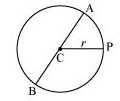Radius of the circle is given =r
C is the center of the circle. AC and CB are the radius of the circle.
So, Diameter AB = AC + CB = r + r = 2r

Question 5
To find sum of three numbers 14, 27 and 13, we can have two ways:
(a) We may first add 14 and 27 to get 41 and then add 13 to it to get the total sum 54 or
(b) We may add 27 and 13 to get 40 and then add 14 to get the sum 54. Thus, (14 + 27) + 13 = 14 + (27 + 13)
This can be done for any three numbers. This property is known as the associativity of addition of numbers. Express this property which we have already studied in the chapter on whole numbers, in a general way, by using variables a, b and c.
14+ 27+13 = (14+27) +13= 41+13=54
14+ 27+13 =14+(27 +13) = 14+40=54
So
(a + b)+c = a +(b + c)

## Exercise 11.3

Question 1
Make up as many expressions with numbers (no variables) as you can from three numbers 5, 7 and 8. Every number should be used not more than once. Use only addition, subtraction and multiplication.
(Hint: Three possible expressions are 5 + (8 − 7), 5 − (8 − 7), (5 × 8) + 7;
make the other expressions.)
Some expressions that can be formed using 5, 8 and 7 are given below.
5 – (8 + 7), 5 + (8 + 7), 5 × (8 + 7), 5 – (8 × 7), 5 + (8 × 7), 5 – (8 – 7)

Question 2
Which out of the following are expressions with numbers only?
(a) $y+ 3$
(b) $(7 \times 20) - 8z$
(c) $5 (21 - 7) + 7 \times 2$
(d) 5
(e) $3x$
(f) $5 - 5n$
(g) $(7 \times 20) - (5 \times 10) - 45 +p$
 a) It carries variable b) It carries variable c) It carries only numbers d) It carries only numbers e) It carries variable f) It carries variable g) It carries variable
Question 3
Identify the operations (addition, subtraction, division, multiplication) in forming the following expressions and tell how the expressions have been formed.
(a) $z+ 1$,$z- 1$,$y+ 17$,$y -17$
(b) $17y,\frac {y}{17},5z$ (c) $2y+ 17$, $2y -17$
(d) $7m$, $-7m+ 3$,$-7m -3$
 a) 1 is added to z, 1 is subtracted from z, 17 is added to y and 17 is subtracted from y. Operations involved are addition, subtraction, addition, subtraction. b) 17 and y are multiplied, y is divided by 17, 5 is multiplied to z. Operations involved are multiplication, division and multiplication c) y is multiplied by 2 and is added to 17, y is multiplied by 2 and 17 is subtracted from the product. Operations involved are multiplication and addition, multiplication and subtraction d) m is multiplied to 7, m is multiplied to -7 and 3 added, m is multiplied to -7 and 3 subtracted. Operations involved are multiplication, multiplication and addition, multiplication and subtraction.

Question 4
Give expressions for the following cases.
(b) 7 subtracted from p
(c) p multiplied by 7
(d) p divided by 7
(e) 7 subtracted from − m (f) − p multiplied by 5
(g) − p divided by 5
(h) p multiplied by – 5
 a) 7 added to p p+7 b) 7 subtracted from p p-7 c) p multiplied by 7 p×7= 7p d) p divided by 7 p/7 e) 7 subtracted from − m -m-7 f) − p multiplied by 5 -p×5=-5p g) − p divided by 5 -p/5 h) p multiplied by – 5 p×(-5)=-5p

Question 5
Give expressions in the following cases.
(b) 11 subtracted from 2m
(c) 5 times y to which 3 is added
(d) 5 times y from which 3 is subtracted
(e) y is multiplied by − 8
(f) y is multiplied by − 8 and then 5 is added to the result
(g) y is multiplied by 5 and the result is subtracted from 16
(h) y is multiplied by − 5 and the result is added to 16
a) $11 + 2m$
b) $2m - 11$
c) $5y+ 3$
d) $5y- 3$
e) $-8y$
f) $-8y+ 5$
g) $16 - 5y$
h) $-5y+ 16$

Question 6
(a) Form expressions using t and 4. Use not more than one number operation. Every expression must have t in it.
(b) Form expressions using y, 2 and 7. Every expression must have y in it. Use only two number operations. These should be different.
a) $t+ 4$,$t- 4$, $4t$,$\frac {t}{4}$, $\frac {4}{t}$, $4 -t$
b)$2y+ 7$,$2y -7$,$\frac {2y}{7}$,$\frac {7}{2y}$,$2 + 7y$### Practice Question

Question 1 What is $\frac {1}{2} + \frac {3}{4}$ ?
A)$\frac {5}{4}$
B)$\frac {1}{4}$
C)$1$
D)$\frac {4}{5}$
Question 2 Pinhole camera produces an ?
A)An erect and small image
B)an Inverted and small image
C)An inverted and enlarged image
D)None of the above